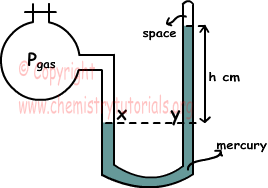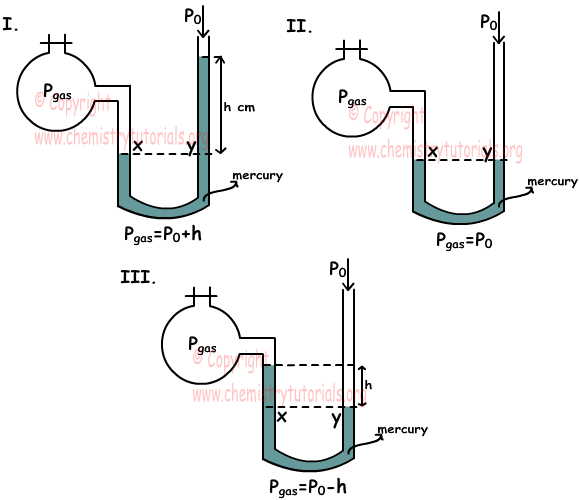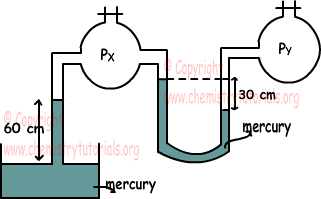EN | ES | DE | NL | RU

## Measuring Pressure of Gas and Manometers with Examples

Manometers with Examples

Pressure of gas in a closed container is equal in everywhere. Manometers are used for measure pressure of gas in closed container. There are two types of manometer, they are in U shape and filled with mercury. If one of the end is open to the atmosphere, we call this type open manometer, and if it is closed, then we cal it closed manometer. We will examine them in detail. Let me begin with closed end manometers.

1. Closed End Manometers:

As you can see from the picture given below one end of manometer is open to gas container and one of them is closed. x and y points in the picture are at same level, thus pressures acting on these points are equal. Pressure at point x is the pressure of gas and pressure at point y is the pressure of mercury at h height.In this system pressure of gas is equal to;

Pgas=h

2. Open End Manometers:

As you can see from the pictures given below, one end of manometer is open to container filled wit gas end one end of it is open to atmosphere. There are three situation we should learn in measuring pressure of gas by the help of atmospheric pressure.In first situation; pressures at points x and y are equal. Px=pressure of gas and Py=h+P0 thus;

Pgas=P0+h

In second situation, pressures at point x and y are also equal and Px=pressure of gas and Py=P0 thus;

Pgas=P0

In third situation; pressures at point x and y are also equal and Px=pressure of gas+h and Py=P0 thus;

Pgas+h=P0

Pgas=P0-h

Example: Find the relation between gases X, Y, Z in the manometers given below.Relation between densities of water and mercury is; dwater<dmercury and P0=75 cm Hg.

X gas in open end manometer;

PX=75 cm Hg+30 cm Hg

Y gas in open end manometer;

PY=75 cm Hg+30 cm H2O

Z gas in closed end manometer;

PZ=75 cm Hg

Since dwater<dmercury pressure of Hg is larger than pressure of H2O. Thus;

PZ<PY<PX

Example: Find pressure of Y gas.(Atmospheric pressure is 75cm Hg)we can write;

PX+60=75

PX=15 cm Hg

And

PY=PX+30=15+30=45

PY=45 cm Hg

The Original Author:

Tags: